初学数模-MATLAB Quick Start！ Part I 原

MATLAB这个语言灰常牛B大家都知道，封装性良好且支持混合编程。比如...OK，现在我们打开MATLAB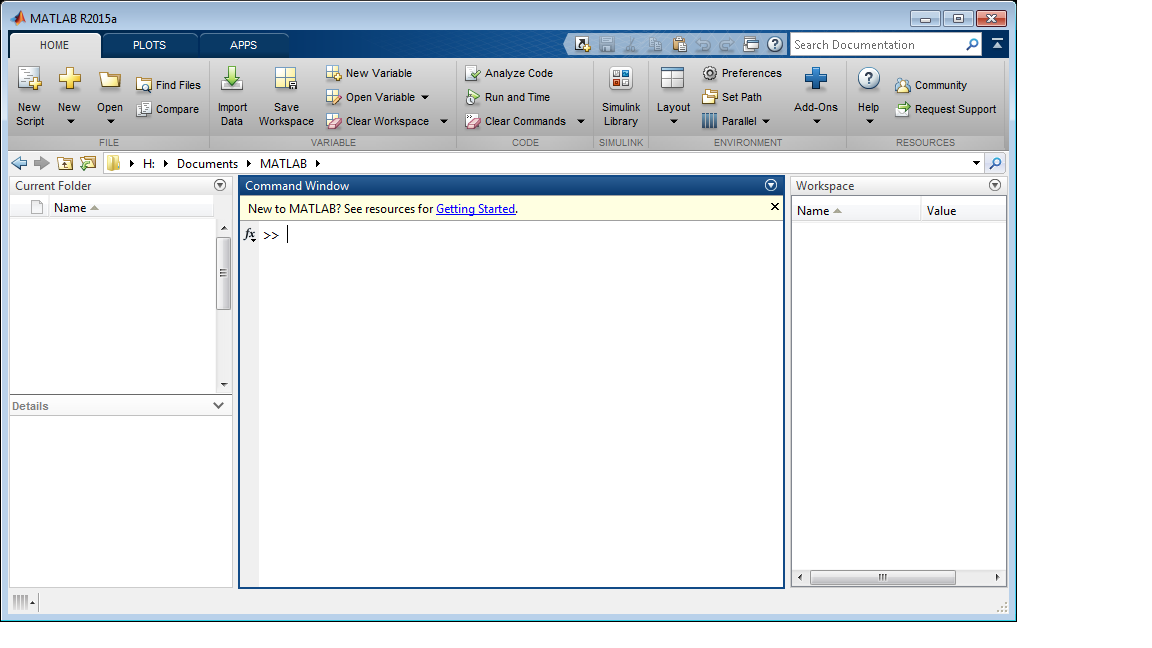Hint: 官方帮助文档很给力！

在MATLAB程序中，由于矩阵运算的重要，我们可以理解为“一切变量皆向量”。

学习语言时，许多时候我们都从Hello World开始，在MATLAB中，这一个程序是这样的：

disp('Hello World!');

恩，确实只需要一行...

按你胃(Anyway)，我们还可以给一个变量赋值

a = 2

还可以让给一个变量赋值为向量

a = [1 2 3 4]

然后，再试一下矩阵

a = [1 2 3; 4 5 6; 7 8 10]

Hint：试一试这些命令，看看程序返回的是什么，再在末尾加上分号(;)试一试！

工作区：当前在工作区（workspace）可用的变量叫做Workspace Varibles。比如，我们运行这两条命令：

A = magic(4);
B = rand(3,5,2);

然后，你就可以在workspace看到它们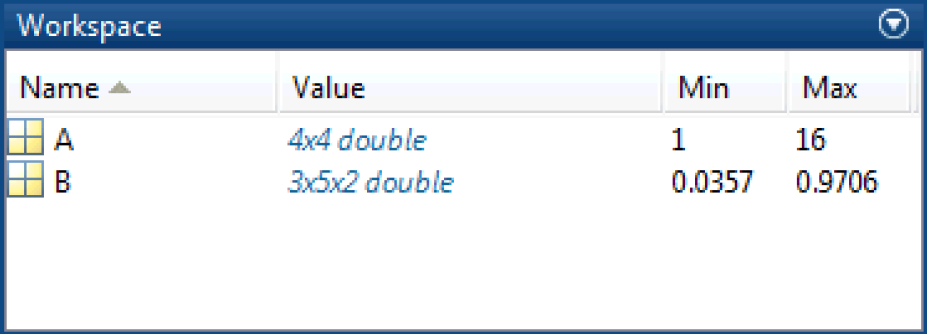另外，我们可以随时使用whos命令来查看当前变量的属性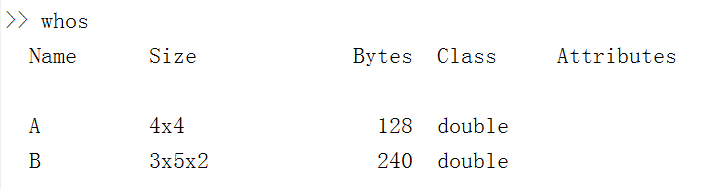还可以这样。。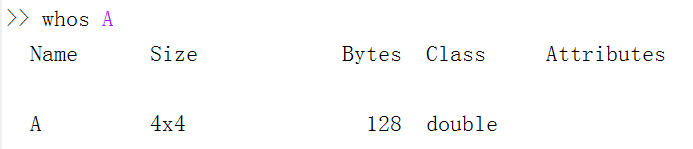MATLAB运算符：在程序中的加减乘除还是用我们熟悉的+、-、*、/，不过在MATLAB中还有一个“左除”，即\，有A\B = B/A。

矩阵乘除运算直接可以用（*/\）这三个符号运算，另外还有点乘（.*）符号表示对应位置相乘，点除、点方(.^n)同理。

不妨自己试一下 B = A.*A

输出格式：试一试输入format long命令，再输入C = B * inv(B)。再把long换成short再试一下

级联：即把两个矩阵连接起来，一般有两种方式——横向（C = [A,B]）和纵向（C = [A;B]）两种方式

注释：打一个百分号，在这一行后面写注释即可

：虚部用i或j表示即可，如：

c = [3+4i, 4+3j; -i, 10j]

矩阵元素的索引：比如A是一个4*4的矩阵，MATLAB提供了两种索引方法：一是A(行,列)，如A(4,2)表示A中第4行第2列的元素；二是A(index)，其中index是按列向下数（和C/C++的按行从左往右刚好相反），如A(3)表示第一列第三行的元素，A(8)表示第4行第2列的元素

Hint：试一试越界的index（如A(17)和A(5,3)），会有什么后果？

那么要同时取几行或几列，要怎么做呢？

这时可以使用冒号：如A(1:3,2)就是矩阵A的1-3行第二列的元素；A(3,:）是矩阵A第三行

冒号还可以这样用：

start:step:end

如D = 0:10:100运行后，D = [0,10,20,30,40,50,60,70,80,90,100]

保存变量到文件：save命令，以.mat为后缀即可，如 save myfile.mat

如何清空：clear命令清空变量，clc命令清空执行过的命令

字符串：MATLAB中的字符串操作和C/C++中类似（就连字符串处理函数的取名也和C/C++差不多。。），这里推荐这篇博文：http://www.cnblogs.com/emanlee/archive/2012/09/13/2683912.html

和普通变量一样，字符串也是“向量”，因此也可以级联。具体命令为：比如此时b = 'tencent'，执行a = ['str',b]；a就变为'strtencent'。

f = 71;
c = (f-32)/1.8;
tempText = ['Temperature is ',num2str(c),'C']

其中num2str函数可以把数字转化成字符串形式。

接下来，我们来看看如何绘制2D和3D的图像吧！

2-D作图利器--plot函数： 先来看个例子，如何绘制y=sin(x)的图像

x = 0:pi/100:2*pi;
y = sin(x);
plot(x,y)

这时候就可以看到图像了。然后可以加上这些命令美化一下

xlabel('x')
ylabel('sin(x)')
title('Plot of the Sine Function')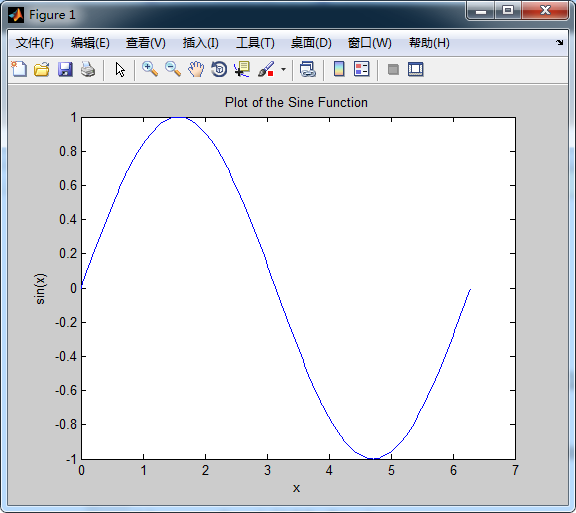Hint：试一下将plot(x,y)改为plot(x,y,'r--')绘制出的图像！

如果要在一张图上绘制多条曲线，则可以用hold on命令

x = 0:pi/100:2*pi;
y = sin(x);
plot(x,y)
hold on %继续在这个Figure上作图
z = cos(x);
plot(x,z,'r--')
xlabel('x')
title('Plot of the Sine and Cosine Function')
legend('sin','cos')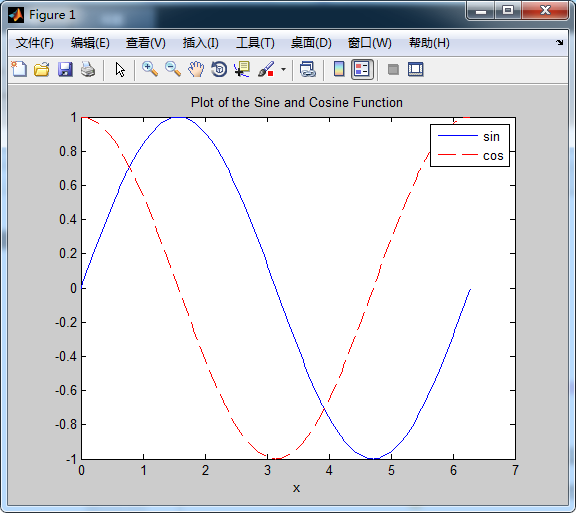3-D作图利器--surf/mesh函数

3D图像可以用meshgrid函数做一个平面，再根据函数方程作图；也可以用参数方程作图。

%做 z=x*e^(-x^2-y^2) 的图像
[X,Y] = meshgrid(-2:.2:2);    %构造平面
Z = X .* exp(-X.^2 - Y.^2);   %构造图形
subplot(2,1,1); surf(X,Y,Z); title('surf');  %使用surf作图
subplot(2,1,2); mesh(X,Y,Z); title('mesh');  %使用mesh作图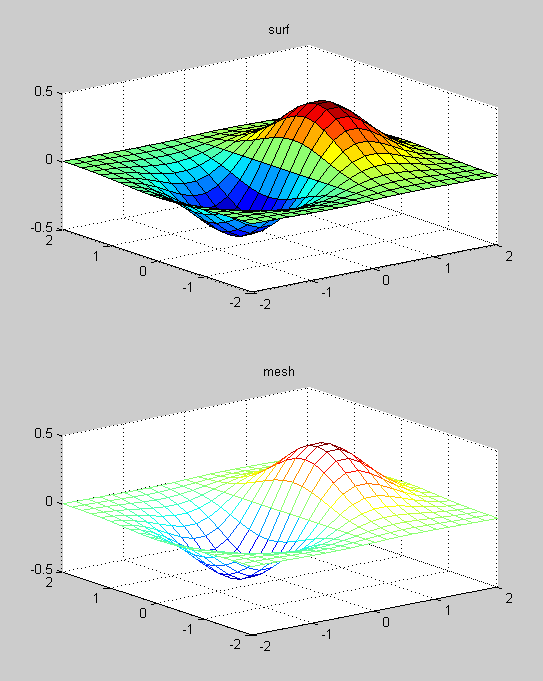上边是用surf函数做的图，下面是用mesh函数做的图，喜欢哪个就用那个啦~

Hint：查阅一下MATLAB官方文档的subplot函数！

然后我们用参数方程做个图：

t = 0:pi/10:2*pi;
[X,Y,Z] = cylinder(4*cos(t));    %即p=4cost
subplot(2,2,1); mesh(X); title('X');
subplot(2,2,2); mesh(Y); title('Y');
subplot(2,2,3); mesh(Z); title('Z');
subplot(2,2,4); mesh(X,Y,Z); title('X,Y,Z');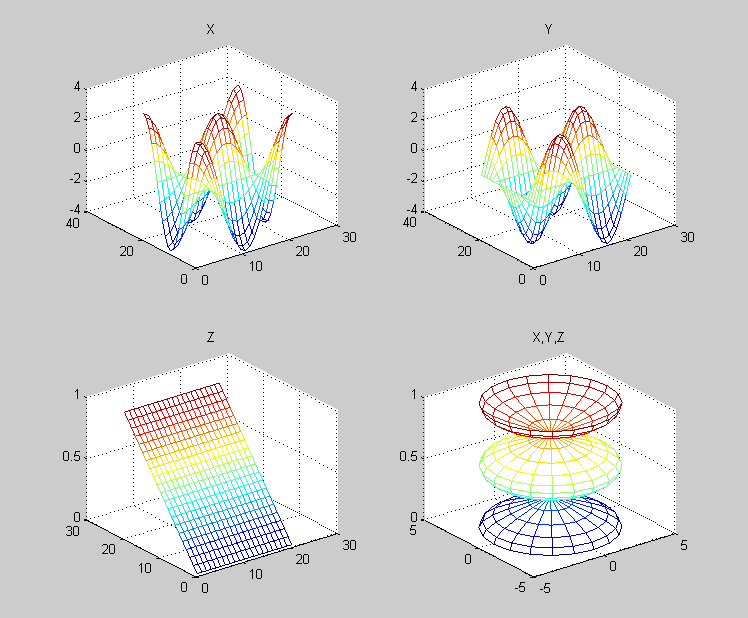MATLAB编程

在MATLAB中编辑文件（如果没有，自动创建.m文件）：edit Calcmean

至于什么循环、条件分支语句，聪明的你肯定一看就能明白：

%Calcmean.m
%计算随机生成数的平均值
clear;            %清除工作区
nsamples = 5;
npoints = 50;
k = 1;

while k<=nsamples  %循环
iterationString = ['Iteration #',int2str(k)];
disp(iterationString)
currentData = rand(npoints,1);
sampleMean(k) = mean(currentData)
k = k+1;
end
%如果去掉 k = k+1; 一句，并把while一行换为for k = 1:nsamples，得到的结果是一样的

overallMean = mean(sampleMean)
if overallMean < .49
disp('Mean is less than expected');
elseif overallMean > .51
disp('Mean is greater than expected');
else
disp('Mean is within the expected range');
end

按下工具条里面绿色的“Run”，就可以运行它了！（PS：感觉跟Python一样一样的！）

Hint：查阅一下官方文档对mean函数的介绍！

Hint：官方文档快速查阅命令有doc和help，如

doc mean
help mean

分别试一下吧！

不知不觉写了一下午，没想到我也能一口气写出3400字的博文，那么MATLAB Quick Start : Part I 就到这里吧！

参考文献：

The MathWorks, Inc. MATLAB® Primer . 2015(24) ： 2-31.不高不富不帅的陈政_

2015/09/15
248
0
【校园团队招兼职程序员，面向广大matlab语言精通者】

2016/05/06
921
0
【招校园兼职程序员】只要你有实力，你肯接单，我们肯给钱！

zhengxinhong
2015/09/20
3
0
matlab关于数据结构算法问题

SevenHao
2015/12/10
159
0
【招校园兼职程序员】只要你有实力，你肯接单，我们肯给钱！

SevenHao
2015/12/05
698
8

popgis

5
0

code-ortaerc

4
0

42
0
Mybatis Plus service

/** * @author beth * @data 2019-10-20 23:34 */@RunWith(SpringRunner.class)@SpringBootTestpublic class ServiceTest { @Autowired private IUserInfoService iUserInfoS......

5
0
php7-internal 7 zval的操作

## 7.7 zval的操作 扩展中经常会用到各种类型的zval，PHP提供了很多宏用于不同类型zval的操作，尽管我们也可以自己操作zval，但这并不是一个好习惯，因为zval有很多其它用途的标识，如果自己...

6
0